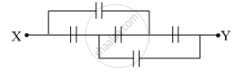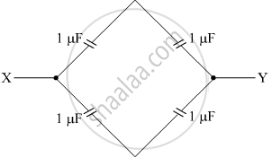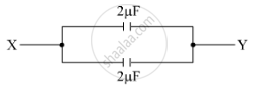Share

# Find the Equivalent Capacitance of the Network - CBSE (Science) Class 12 - Physics

#### Question

Find the equivalent capacitance of the network shown in the figure, when each capacitor is of 1 μF. When the ends X and Y are connected to a 6 V battery, find out (i) the charge and (ii) the energy stored in the network.#### Solution

The equivalent circuit is given below.There are two capacitors in one branch in series. So, the equivalent capacitance of one branch will be

1/1+1/1=2muF

The arrangement will be further reduced to the form given below.Now, both the capacitors are in parallel, so the equivalent capacitance will be

2+2=4μF

Therefore, the equivalent capacitance is 4 μF.

(i)

Voltage, V = 6 V

The charge in the network is given by

q=CV

Here, C is the equivalent capacitance.

Now,q=4×106×6

=24×106 C=24 μC

(ii)

The energy stored in the network is given by
E=1/2CV2

=12×4×106×(6)2

=12×4×36×106

=72×106 J

=72 μJ

Is there an error in this question or solution?

#### Video TutorialsVIEW ALL 

Solution Find the Equivalent Capacitance of the Network Concept: Capacitors and Capacitance.
S